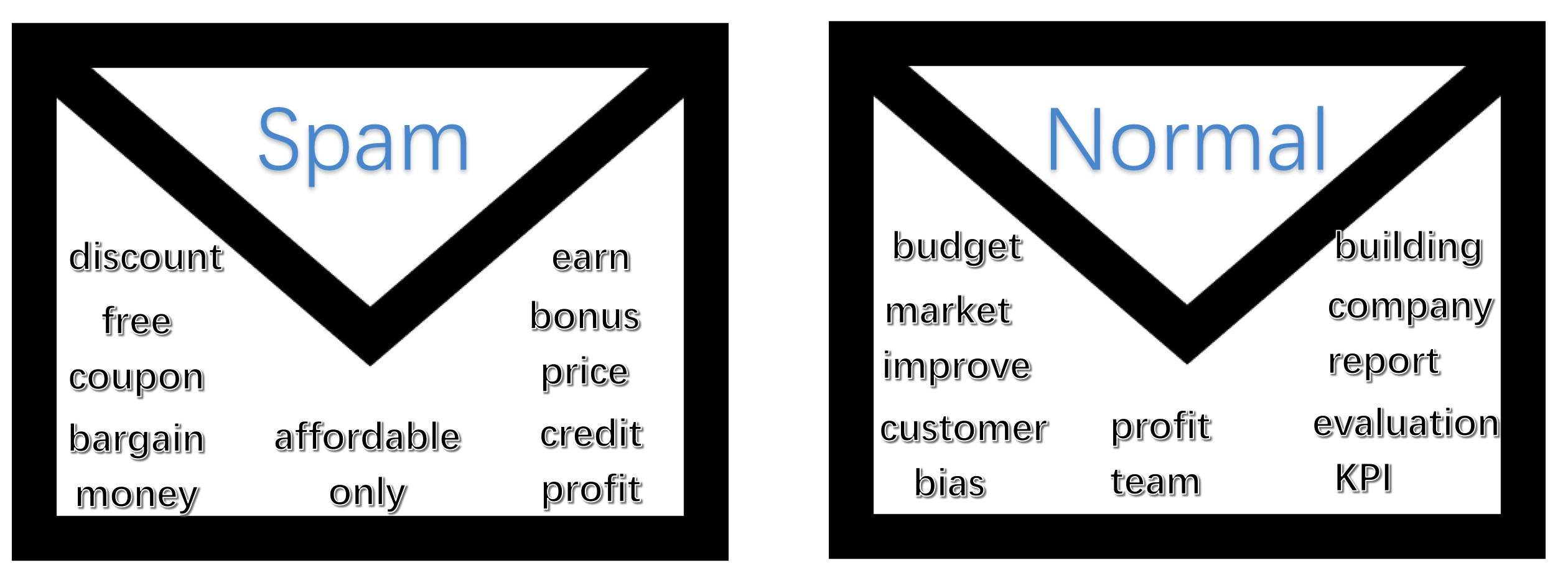### Introduction

You are sitting in front your sceen, annoyed by a bunch of spam mails. You wonder if there are any appoaches to get rid of so much many offended emails. Last time you doped out a extremely good idea. You set a series of words to identify those emails: every mail invovled by words “coupon” was trown to trash. However, on one hand, there were only about 10% spam including “coupon”, one the other hand, you had trashed two significant emails, due to which, you lost two business valued about two million dollars. The thing was that, your inbox seems being overrun by those spams. Who can rescue you from endless deleting spams everyday?

### Intuition

Actually, you were close to the answer when you were putting all emails to trash which included the word “coupon”. Today, we learn about an efficient method to solve the problem systematically.Maybe we can search through internet, find all words which are about advertisment in email. You can restrict that if and only if those which includes more than 4 word in the list of spam words can be put into trash can. Maybe finally you can design a rule system to recoginize those spams without miss important ones. But it seems so boring a job to do this, moreover, it may be not personalized. If some those who are working for saling discount stuffs, he/she may find it doesn’t work through applying your effective rules. How about thinking of probablity of those words emerge in all your inbox. Some words such as “coupon” may contribute more but not entirety, simultaneously, affordable may contribute less but not none. Notice that profit emerge in spam emails and normal emails both sometimes.
We let $y=0$ denote an normal email while $y=1$ the opposite. And if a word such as “coupon” emerges in a mail, we set $coupon=1$, otherwise $coupon=0$. Suppose you have an email, we define the probability to :
\begin{align}
&p1=p(y=0|free=1, discount=1, affordable=1, customer=0, KPI=0, budget=0,…,bias=0)=?\
&p2=p(y=1|free=1, discount=1, affordable=1, customer=0, KPI=0, budget=0,…,bias=0)=?
\end{align}
Our aim is to decide which is bigger $p1$ or $p2$. Let’s describe the problem as followed:

We want to decide the probality of a mail spam or normal when word “free” is in the mail, “discount” is in the mail, “affordable” is in the mail, customer is not in the mail, …, “bias” is not.

The description above is only about one email. For some other emails, maybe “free” and “discount” both did not emerge at all.

### Definitioin

To generalize the problem, we let $x_i$ denote a word in emails. Suppose we now know all words in your inbox, say 10 thousand words. Then we have $x_1$ denote if “free” is in a mail, $x_1=0$ denotes negative while $x_1=1$ denotes positive. So we have $x_1,x_2,x_3,…,x_{10000}$, which denotes the status of each word in a mail. Then the problem is transferred as followed:
\begin{align}
&p1=p(y=0|x_1=1, x_2=1, x_3=1, x_4=0, x_5=0, x_6=0,…,x_{10000}=0)=?\
&p2=p(y=1|x_1=1, x_2=1, x_3=1, x_4=0, x_5=0, x_6=0,…,x_{10000}=0)=?
\end{align}
Suppose we have N words in your inbox, then we want to decide:
\begin{align}
&p1=p(y=0|x_1, x_2,…,x_{N})=?\
&p2=p(y=1|x_1, x_2,…,x_{N})=?
\end{align}
So how to sovle the probability problem?

### Naive Bayes

Recall Bayes rules:
\begin{equation}
p(y|x)=\frac{p(x,y)}{p(x)}=\frac{p(x|y)p(y)}{p(x)}
\end{equation}
we apply the equation to our problem, then we have:
\begin{align}
&p(y=1|x_1,x_2,…,x_N)\
& =\frac{p(x_1,x_2,…,x_N,y=1)}{p(x_1,x_2,…,x_N)}\
& =\frac{p(x_1,x_2,…,x_N|y)p(y=1)}{p(x_1,x_2,…,x_N)}\
\
&p(y=0|x_1,x_2,…,x_N)\
& =\frac{p(x_1,x_2,…,x_N,y=0)}{p(x_1,x_2,…,x_N)}\
& =\frac{p(x_1,x_2,…,x_N|y)p(y=0)}{p(x_1,x_2,…,x_N)}
\end{align}
Notice that {p(x_1,x_2,…,x_N) is positive, and a constant as well. so our aim is transferred to:
\begin{align}
&Max(p(y=1|x_1,x_2,…,x_N), p(y=0|x_1,x_2,…,x_N)\
&=Max(p(x_1,x_2,…,x_N|y=1)p(y=1),p(x_1,x_2,…,x_N|y=0)p(y=0)
\end{align}

First of all, we talk about how to get $p(y=0)$ and $p(y=1)$. We have $N=10000$, suppose there are $900$ spams and $91000$ normal emails, then:
\begin{align}
&p(y=0)=\frac{count(spam\ email)}{count(all\ emails)}=\frac{900}{10000}=0.09\
&p(y=1)=\frac{count(normal\ email)}{count(all\ emails)}=\frac{9100}{10000}=0.91
\end{align}
And right now our task left is to compute $p(x_1,x_2,…,x_N|y=0)$ and $p(x_1,x_2,…,x_N|y=1)$, that means we want to know if a mail is a normal one or not, what is the probability of the combination of these $N=10000$ words. In other word, $x_1=0\ or\ 1$,$x_2=0\ or\ 1$ and so on. So we have to compute $22^{10000}=21.995*10^{3010}$ probabilities under our circumstance. It seems the scale is so large that we can not handle it. So we have an extremely adventurous assumption:

Each x in an email only can be decided by y.

Then we have:
\begin{align}
p(x_1,x_2,…,x_N|y=0)=p(x_1|y=0)\cdot p(x_2|y=0)\cdots p(x_N|y=0)\
p(x_1,x_2,…,x_N|y=1)=p(x_1|y=1)\cdot p(x_2|y=1)\cdots p(x_N|y=1)
\end{align}
Now, we just need compute 2*10000 probabilities, it is surely a mission possible now. So how to compute $p(x_i=0\ or\ 1|y=0\ or\ 1)$? Take word “free” for example, we want to compute:
\begin{align}
&p(free=0|y=0)\
&=\frac{p(free=0, y=0)}{p(y=0)}\
&=\frac{count\ of\ emails\ have\ no\ word\ “free”\ in\ normal\ emails}{count\ of\ normal\ emails}\
&p(free=0|y=1)\
&=\frac{p(free=0, y=1)}{p(y=1)}\
&=\frac{count\ of\ emails\ have\ no\ word\ “free”\ in\ spams}{count\ of\ spam\ emails}
\end{align}
As long as we have computed all of these values, we can use the equation to decide which email should be trashed. Suppose we have computed the probabilites of $p1$ and $p2$:
\begin{align}
&p1\triangleq p(x_1,x_2,…,x_N|y=1)p(y=1)\triangleq p(y=0)\cdot \Pi_{i=1}^N p(x_i|y=0)=0.00091\
&p2\triangleq p(x_1,x_2,…,x_N|y=0)p(y=0)\triangleq p(y=1)\cdot \Pi_{i=1}^N p(x_i|y=1)=0.00000032
\end{align}
Then we consider that the email is more of a spam mail.

### Summary

Today we have talked about how to run a Naive Bayes algorithm to decide if an email is a spam. We suppose each word in a mail is no of business of the other, which is a simple assumption named conditional independence assumption but not the reality(e.g. “coupon” maybe emerges with “save” and “money” due to their inner association). However, the algorthm is very effective.

### Future Thinking

Suppose if a word “wooooo” haven’t emerged in inbox, then the probability will reach $p1=p2=0$, how to solve it?
Suppose you have a task to differiate oranges from apples and pears using color and shape, how to design the algorithm?
suppose x is continuous rather than discrete, Naive Bayes still works or not?